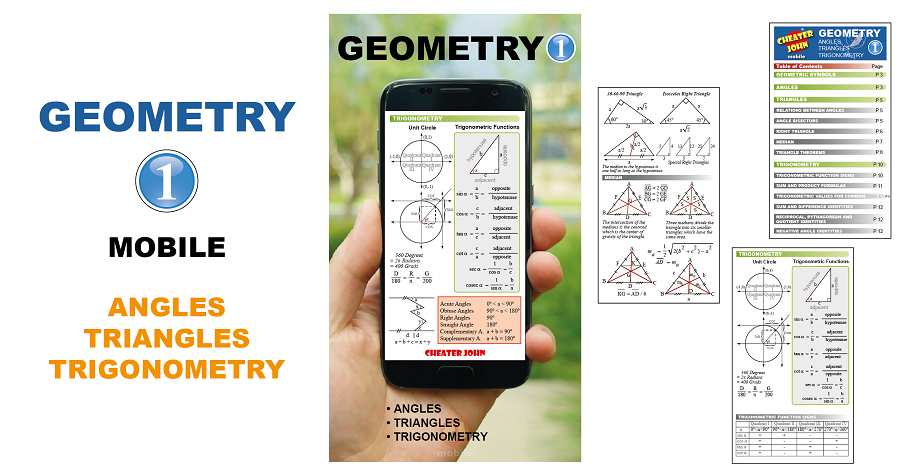# Geometry Formulas and Equations 1 MobileGeometry formula reference / cheat sheet. For everyday use at school, work and home.

Designed for mobile phones.
Angles
Type Of Angles

Triangles
Relations Between Angles
Interior Angles, Exterior Angles
Angle Bisectors
Median
Triangle Theorems
Right Triangle
Similarity
Interior Angle Bisector Theorem, Exterior Angle Bisector Theorem
Ceva’s Theorem, Stewart’s Theorem, Basic Proportionality Theorem, Menelaus’ Theorem, Carnot’s Theorem

Trigonometry
Unit Circle
Trigonometric Functions
Sum and Product Formulas
Sum and Difference Identities
Reciprocal, Pythagorean and Quotient Identities
Negative Angle Identities
Trigonometric Function Signs
Trigonometric Values for Common Angles